# Lacunary trigonometric series

A series of the form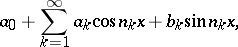(1)

with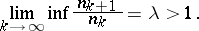In 1872 K. Weierstrass presented a continuous nowhere-differentiable function by means of a series of type (1). In 1892 J. Hadamard applied the series (1), calling them lacunary, to the study of analytic continuation of functions. A systematic study of lacunary trigonometric series began with a paper by P. Fatou in 1906, in which he proved that everywhere convergence of a lacunary trigonometric series forimplies that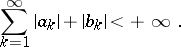(2)

Lacunary trigonometric series have properties essentially different from those of general trigonometric series. For example, A.N. Kolmogorov, having constructed the first example (1923) of a summable function with Fourier series diverging almost-everywhere, proved in 1924 that a lacunary Fourier series converges almost-everywhere; A. Zygmund proved (1948) that if the sums of two lacunary trigonometric series coincide on a set of positive measure, then these series are identical. For many applications of lacunary trigonometric series the dependence of properties of the series (1) on its coefficients, discovered by Zygmund in the 1930's, is important. Thus, if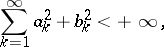(3)

then the series (1) is the Fourier series of a functionbelonging to all spaces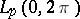,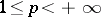, hence it converges almost-everywhere. There are constants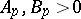depending only onandsuch that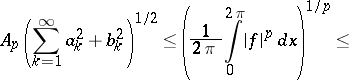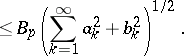If (3) is not satisfied, then the series (1) diverges almost-everywhere, and, moreover, almost-everywhere it cannot be summed by any Toeplitz method (cf. Toeplitz matrix) (and is therefore not a Fourier series). If the series (1) converges at every point of some interval, then (2) is satisfied. If the coefficients of the series (1) are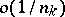, then its sum is a continuous smooth function, differentiable at just those points at which the formally differentiated series of (1) converges.